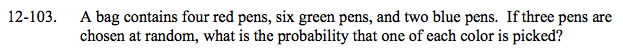### Home > A2C > Chapter 12 > Lesson 12.2.1 > Problem12-103

12-103.

A bag contains four red pens, six green pens, and two blue pens. If three pens are chosen at random, what is the probability that one of each color is picked? Homework Help ✎$\frac{_{4}C_{1}\cdot(?)(?)}{_{12}C_{3}}$

$\frac{12}{55}$